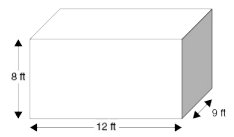# An interior decorator plans to wall-paper three walls of a bedroom. In the following depiction of the room, the shaded wall will not be wallpapered.Wallpaper is measured by the square yard. Each single roll of wallpaper contains 4 square yards of wallpaper. What is the LEAST number of wallpaper rolls that must be purchased to cover the three walls?

Explanation

Two of the walls are 12 feet by 8 feet, and one wall is 9 feet by 8 feet. Find the area of the three walls:
$$2 \times 12 \text { feet } \times 8 \text { feet }=192 \text { square feet }$$
$$1 \times 9 \text { feet } \times 8 \text { feet }=72 \text { square feet }$$
The total area of the three walls is 192 square feet 72 square feet = 264 square feet. Since wallpaper is sold by the square yard, it is necessary to convert from square feet to square yards. Since 9 square feet = 1 square yard, 264 square feet / 9 square feet = 29(1/3)square yards.
From the information provided, you know that each wallpaper roll contains 4 square yards of wallpaper. So 29(1/3) square yards divided by 4 = 7(1/3) rolls. Since wallpaper is only sold by the ic full roll, you will need to purchase 8 rolls.